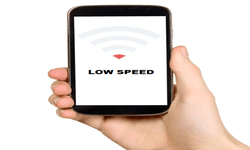# LTE Reference Sensitivity Calculation, How it depends on SNR, why throughput decrease at the Cell edge?

The LTE reference sensitivity power level is the minimum mean power received at the antenna connector at which a throughput shall be ≥ 95% of the maximum throughput for a specified reference measurement channel.

In layman’s terms, it means that the minimum power level of LTE signal that we apply at the antenna connector of base station / UE, and till the throughput of that device is greater than or equal to 95%, is known as reference sensitivity level.

#### Required SNR

Signal to Noise Ratio (SNR) is the SNR is defined as the ratio of signal power to the noise power, often expressed in decibels. Here the Required SNR means that minimum level of SNR required to decode the type of LTE signal. LTE signal could be of different Code rate / Modulation etc. So for each type of LTE signal, required SNR may vary.

As one can see in the below table, the SNR required for different type of LTE signal.#### Thermal Noise

Johnson–Nyquist noise (sometimes thermal, Johnson or Nyquist noise) is unavoidable, and generated by the random thermal motion of charge carriers (usually electrons), inside an electrical conductor, which happens regardless of any applied voltage.

Thermal noise is approximately white, meaning that its power spectral density is nearly equal throughout the frequency spectrum.

Thermal noise in a 50 Ω system at room temperature is -174 dBm / Hz.

#### Noise Figure

Noise figure (NF) is a measure of degradation of the signal-to-noise ratio (SNR), caused by components in a signal chain. It is a number by which the performance of an amplifier or a radio receiver can be specified, with lower values indicating better performance.

NF is the ratio of input SNR to the output SNR of a device or receiver chain. It is expressed in dB.

Typically value of NF for a LTE receiver chain is 4 to 5dB.

#### Reference Sensitivity (S) Calculation

Now, let’s put together all the terms discussed above in reference sensitivity formula.

S = Thermal Noise + NF + 10*Log(Bandwidth) + SNR

Suppose, LTE signal is 5MHz of bandwidth, with QPSK modulation and Code rate of 1/3.

From SNR table, corresponding required SNR is -1dB.

Suppose, NF of LTE receiver chain is around 5dB.

S = -174dBm/Hz + 5dB + 10*Log(5MHz) + (-1dB)

= -174 + 5 + 67 -1

= -103dBm

Now if you increase the modulation to be 16QAM with code rate of 2/3, the required SNR becomes 11.3dB (as per table in SNR section).

So, S = -174 + 5 + 67 + 11.3

= -90.7dBm

As we see, with higher modulation, the sensitivity level decreases. So at the cell edges, where already signal level is low, thus UEs are assigned lower modulations to maintain the connectivity with the base station and thus with lower modulation at the cell edges, the throughput of UE also decreases.

This is a very basic calculation of reference sensitivity. In actual system, there are many other factors that will be affecting the reference sensitivity levels, which we will be discussing in coming posts.